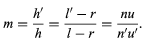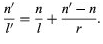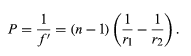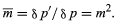Courses

# The Optical Invariant Physics Notes | EduRev

## Physics : The Optical Invariant Physics Notes | EduRev

The document The Optical Invariant Physics Notes | EduRev is a part of the Physics Course Basic Physics for IIT JAM.
All you need of Physics at this link: Physics

Magnification:

It is frequently as important to determine the size of an image as it is to determine its location. To obtain an expression for the magnification—that is, the ratio of the size of an image to the size of the object—the following process may be used: If an object point B lies to one side of the lens axis at a transverse distance h from it, and the image point B′ is at a transverse distance h′, then B, B′, and the centre of curvature of the surface, C, lie on a straight line called the auxiliary axis. Then, by simple proportion,Hence,

h'n'u' = hnu (7) and the product (hnu) is invariant for all the spaces between the lens surfaces, including the object and image spaces, for any lens system of any degree of complexity. This theorem has been named after the French scientist Joseph-Louis Lagrange, although it is sometimes called the Smith-Helmholtz theorem, after Robert Smith, an English scientist, and Hermann Helmholtz, a German scientist; the product (hnu) is often known as the optical invariant. As it is easy to determine the quantities h, n, and u for the original object, it is only necessary to calculate u′ by tracing a paraxial ray in order to find the image height h′ for any lens. If the lens is used in air, as most lenses are, the refractive indices are both unity, and the magnification becomes merely m = u/u'.

The thin lens:

In a thin lens such as a spectacle, the two principal planes coincide within the lens, and then the conjugate distances p and p′ in the formula above become the distances of object and image from the lens itself.

The focal length of a thin lens can be computed by applying the surface-conjugate formula (6) to the two surfaces in succession, writing the l of the first surface as infinity and the l of the second surface equal to the l′ of the first surface. When this is done, the lens power (P) becomes(6)Chromatic aberration:

Because the refractive index of glass varies with wavelength, every property of a lens that depends on its refractive index also varies with wavelength, including the focal length, the image distance, and the image magnification. The change of image distance with wavelength is known as chromatic aberration, and the variation of magnification with wavelength is known as chromatic difference of magnification, or lateral colour. Chromatic aberration can be eliminated by combining a strong lens of low-dispersion glass (crown) with a weaker lens made of high-dispersion (flint) glass. Such a combination is said to be achromatic. This method of removing chromatic aberration was discovered in 1729 by Chester Hall, an English inventor, and it was exploited vigorously in the late 18th century in numerous small telescopes. Chromatic variation of magnification can be eliminated by achromatizing all the components of a system or by making the system symmetrical about a central diaphragm. Both chromatic aberration and lateral colour are corrected in every high-grade optical system.

Longitudinal Magnification:

If an object is moved through a short distance δp along the axis, then the corresponding image shift δp′ is related to the object movement by the longitudinal magnification (m). Succinctly,in which m is the lateral magnification. The fact that the longitudinal magnification is equal to the square of the transverse magnification means that m is always positive; hence, if the object is moved from left to right, the image must also move from left to right. Also, if m is large, then m is very large, which explains why the depth of field (δp) of a microscope is extremely small. On the other hand, if m is small, less than one as in a camera, then m is very small, and all objects within a considerable range of distances (δp) appear substantially in focus.

Image of a tilted plane:

If a lens is used to form an image of a plane object that is tilted relative to the lens axis, then the image will also be tilted in such a way that the plane of the object, the plane of the image, and the median plane of the lens all meet. This construction can be derived by the use of the lateral and longitudinal magnification relations just established above. With a tilted object the magnification at any point is given by the ratio of the distances of image and object from the lens at that point in the image, and, consequently, m varies progressively from one end of the image to the other. This arrangement is frequently used in view cameras equipped with “swings” to increase depth of field and in enlargers to rectify the convergence of parallel lines caused by tilting the camera, for example, in photographing tall buildings. The rule finds extensive application in photo-grammetry and in the making of maps from aerial photographs.

Offer running on EduRev: Apply code STAYHOME200 to get INR 200 off on our premium plan EduRev Infinity!

## Basic Physics for IIT JAM

109 videos|157 docs|94 tests

,

,

,

,

,

,

,

,

,

,

,

,

,

,

,

,

,

,

,

,

,

;